CAT  >  Test: CAT Quantitative Aptitude- 3

# Test: CAT Quantitative Aptitude- 3

Test Description

## 22 Questions MCQ Test CAT Mock Test Series | Test: CAT Quantitative Aptitude- 3

Test: CAT Quantitative Aptitude- 3 for CAT 2022 is part of CAT Mock Test Series preparation. The Test: CAT Quantitative Aptitude- 3 questions and answers have been prepared according to the CAT exam syllabus.The Test: CAT Quantitative Aptitude- 3 MCQs are made for CAT 2022 Exam. Find important definitions, questions, notes, meanings, examples, exercises, MCQs and online tests for Test: CAT Quantitative Aptitude- 3 below.
Solutions of Test: CAT Quantitative Aptitude- 3 questions in English are available as part of our CAT Mock Test Series for CAT & Test: CAT Quantitative Aptitude- 3 solutions in Hindi for CAT Mock Test Series course. Download more important topics, notes, lectures and mock test series for CAT Exam by signing up for free. Attempt Test: CAT Quantitative Aptitude- 3 | 22 questions in 40 minutes | Mock test for CAT preparation | Free important questions MCQ to study CAT Mock Test Series for CAT Exam | Download free PDF with solutions
 1 Crore+ students have signed up on EduRev. Have you?
Test: CAT Quantitative Aptitude- 3 - Question 1

### The average age of a group of travellers is 25 years. For a certain trip to Australia, the average age has to be a maximum of 24 years, so they included A in their group to satisfy the criteria. For a certain trip to South Africa, the average age of the group has to be a minimum of 26 years. To satisfy this, the original group (excluding A) included B in their group. What is the average of the highest possible age of A and the lowest possible age of B?

Detailed Solution for Test: CAT Quantitative Aptitude- 3 - Question 1

Let the number of people in the group be n
For the maximum possible age of A,
24(n+1) = 25n + A
A = 24 - n
For minimum possible age of B,
26(n+1) = 25n + B
B = n + 26
Hence, average = (24 - n + n + 26)/2 = 25 years
Option (D)

Test: CAT Quantitative Aptitude- 3 - Question 2

### A bag contains Rs. 1, 50 p and 25 p coins. The number of 25 p coins is numerically equal to the total value of all the 50 p coins (in Rs). Aso, the total amount in the bag is equivalent to one thousand 25 p coins. The number of Rs. 1 coins is 14 less than the total value of all 25 p coins(in Rs). What is the total value of all the 50 p coins as a percentage of the total amount of money present in that bag?

Detailed Solution for Test: CAT Quantitative Aptitude- 3 - Question 2

Let the number of 50 p coin be n.
Hence value = n/2.
Number of 25 p coins = n/2.
Number of Rs 1 coins = n/8 - 14
Hence,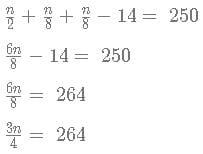n = 352
Hence, required percentage = 176/250 x 100 = 70.40 %

*Answer can only contain numeric values
Test: CAT Quantitative Aptitude- 3 - Question 3

### A mixed fraction is as follows: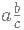where a>=0, b>0, c>0 and a,b,c are whole numbers. Also, b/c cannot be further reduced, that is, b and c are co-prime, and b<c. It is known that b is 20% of a and c is 30% of a. How many such mixed fractions are possible?

Detailed Solution for Test: CAT Quantitative Aptitude- 3 - Question 3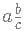b = 20% of a = a/5
c = 30% of b = 3b/10
So, 'a' must be a multiple of 10.
Let us assume that a = 10n, where n = 1, 2, 3 etc
when a = 10,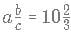When a = 20, b = 4 and c = 6, this is not possible, since b and c should be co-prime
Similarly, when a = 30, b = 6 and c = 9, again they are not co-prime,
So, we can infer when a = 10n, b = 2n and c = 3n.
Hence, only n the case when n = 1, they satisfy the condition.
Hence, 1 fraction is possible only.

Test: CAT Quantitative Aptitude- 3 - Question 4

In Cracku, 60% of the enrolled students read newspaper A, 50% of the enrolled students read newspaper B, 30% of the enrolled students read newspaper C, 40% of the enrolled students read newspaper D. While a particular student may read more than one newspaper, each student must read at least one newspaper. It is known that no one reads 2 or 3 newspapers, which of the following is a possible number of enrolled students in Cracku?

Detailed Solution for Test: CAT Quantitative Aptitude- 3 - Question 4

I + II + III + IV = 100%
I + 2 II + 3 III + 4 IV = 60 + 50 + 30 + 40 = 180%
Hence, the additional 80% should be distributed among II + 2 III + 3 IV.
It is mentioned that II = 0, III = 0
Hence, IV = 80/3%
Now, IV has to be natural number since it is the number of students.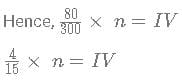Hence, n must be a multiple of 15.
But, we have not yet considered the people who only follow one newspaper.
People who only follow A =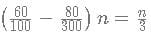People who only follow B =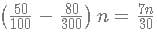People who only follow C =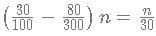People who only follow D =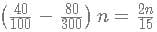Hence, n must be divisible by 30 as well.
Hence, 30030 is the only possible alternative.

Test: CAT Quantitative Aptitude- 3 - Question 5

A function f(x) is defined as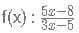fn (x) is defined as f(f(...n times f(x))))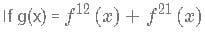What is value of [g(12)]. [x] represents the greatest integer less than or equal to x

Detailed Solution for Test: CAT Quantitative Aptitude- 3 - Question 5

Let us start by calculating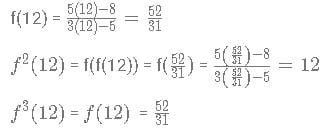We observe that when n is odd  then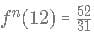when n is even  then fn (12) = 12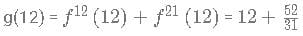[g(12)] = 13

Test: CAT Quantitative Aptitude- 3 - Question 6

If a polynomial function exists such that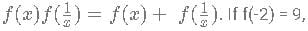what is the absolute value of f(4)?

Detailed Solution for Test: CAT Quantitative Aptitude- 3 - Question 6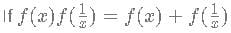then f(x) has to be of form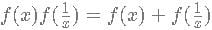this can be written as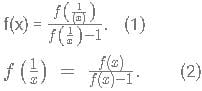Multiplying 1 and 2 we get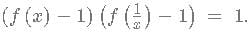Assuming f(x)-1 = g(x) gives us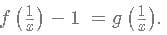As per the conditions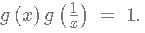Since f(x) is a polynomial function g(x) is a polynomial function.
g(x) can be satisfied only by ± xn
Hence f(x) = 1 + xn
f(-2) = 1 + (−2)n = 9 or + (−2)n = 8
Thus n=3 and function has to be 1 - x3
f(4) = 1 - 43 = 1 - 64 = -63
Modulus value = 63

*Answer can only contain numeric values
Test: CAT Quantitative Aptitude- 3 - Question 7

Let p be sum of all the values of kk which satisfy the equation:
5{k}+2 = 2[k-1]
What is the value of [2p-1]?
Here {x} denotes the fractional part of x and [x] denotes the greatest integer less than or equal to x

Detailed Solution for Test: CAT Quantitative Aptitude- 3 - Question 7

5{k}+2 = 2[k-1]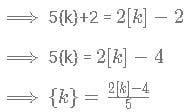We know, 0 < {k} < 1
Least value of [k] which satisfies is [k] = 2 at which {k} =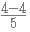0. Thus k ={k}+[k] = 2.0
When [k]=3,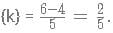Thus k ={k}+[k] =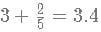When [k]=4, {k} =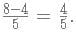Thus k ={k}+[k] =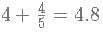When [k]=5, {k} =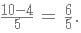Which is not possible
Sum of all possible value of k = 2+3.4+4.8 = 10.2 which is equal to p
2p - 1 = 2 x 10.2 - 1 = 20.4 - 1 = 19.4
[2p-1] = 19

Test: CAT Quantitative Aptitude- 3 - Question 8

Find the minimum value of the following function:
f(x) = max (x - 5x + 6,x2)

Detailed Solution for Test: CAT Quantitative Aptitude- 3 - Question 8

f(x) = max(x2 - 5x + 6,x2)
The difference between the 2 expressions is -5x + 6.
Whenever this part is greater than or equal to 0, f(x) is the first function, else, the second function.
-5x + 6 >= 0
x <= 6/5
Hence, for x <= 6/5, f(x) = x2 - 5x + 6
For x > 6/5, f(x) = x2
Since they both are quadratic, they will have the same value at x = 6/5.
Also, at x < 6/5, f(x) is the left side of a U - shaped quadratic curve(part of the quadratic curve before the leftmost root)
At x >= 6/5, f(x) is the right side of a U - shaped quadratic curve (part of the quadratic curve after the rightmost root)
Hence, the minimum value of the function is at x = 6/5.
Value = x2 = 36/25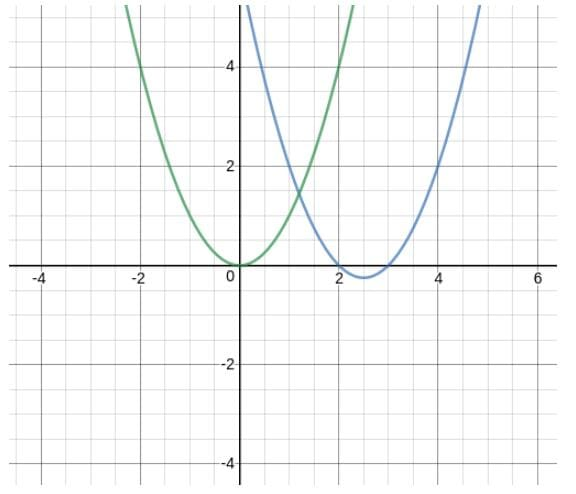Test: CAT Quantitative Aptitude- 3 - Question 9

10 spheres of radius "R" are melted into making right cones of radius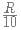and height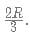What is approximate change in the curved surface area?

Detailed Solution for Test: CAT Quantitative Aptitude- 3 - Question 9

Initial volume of 10 Spheres =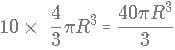Let N be the number of cones which can be made by  melting the sphere.
Volume of a cone =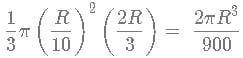As per question =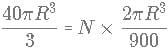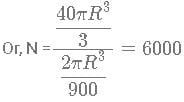Initial curved surface area = 10×4πR2 = 40πR2 ~ 125.6R2
Curved surface of the cone = πrl where r is radius of the base and l is the slant height of the cone.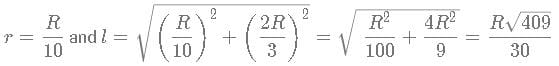Total curved surface area for the Cone =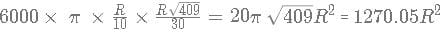Change in Curved Surface Area = 1270.05R2 - 125.6R2 =1144.45R2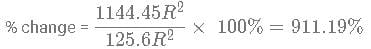Test: CAT Quantitative Aptitude- 3 - Question 10

2 circles of the same radius are on a 2-D plane that exists such that the centre of one circle lies on the circumference of another circle. A quadrilateral is constructed by taking the two points of intersection of the circles and their centres. What is the ratio of the area of the quadrilateral to the area of 1 circle?

Detailed Solution for Test: CAT Quantitative Aptitude- 3 - Question 10In the above figure, we need to find the Area of BO'CO
Since B and C are on the circumference of the circle, BO=CO=BO'=CO' =r . Also OO' =r
We can say that BOO' and COO' are 2 equilateral triangles with sides r
Thus area of BO'CO = area BOO' + area COO'
Thus area of BO'CO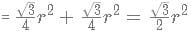Area of 1 circle = πr2
Ratio =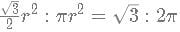Test: CAT Quantitative Aptitude- 3 - Question 11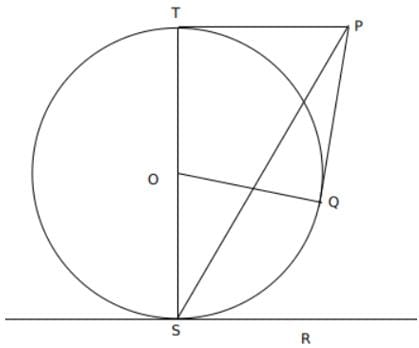In the above figure, PT and RS are 2 parallel lines which are tangents to the circle with centre O. PQ is also a tangent to the circle
∠ PSO = 30º and PT = 6 units. What is the value of the area of PTOQ?

Detailed Solution for Test: CAT Quantitative Aptitude- 3 - Question 11

Let the diameter of the circle be 2r
△SPT is a right-angle triangle.
tan(30º) = 6/2r
1/√3 = 3/r
or r = 3√3. Since PT and PQ are the tangents from same point P, PT = PQ
Area PTOQ = Area PTO + Area PQO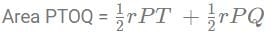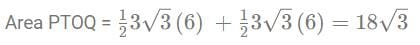Test: CAT Quantitative Aptitude- 3 - Question 12

How many positive integral pairs (x, y) exists such that 4 < ∣x − 5∣ × ∣y − 7∣ < 7

Detailed Solution for Test: CAT Quantitative Aptitude- 3 - Question 12

Since it is given that xx and yy are integers then |x - 5|×|y - 7| also needs to be an integer.
Thus |x − 5| × |y − 7| = 5 or 6
Case I: |x − 5| × |y − 7| = 5
a) |x - 5| = 5 and |y - 7| = 1 It gives x = 0 or 10 and y = 6 or 8. (10, 6) and (10, 8) are only solution as we are given that x, yx, y are positive
b) |x - 5| = 1 and |y - 7| = 5. It gives x = 6 or 4 and y = 12 or 2. Total of 4 such pairs are possible
Total 6 such pairs possible for case I
Case II: |x − 5| × |y − 7| = 6
a) |x - 5| = 1 and |y - 7| = 6. It gives x = 4 or 6 and y = 1 or 13 Thus 4 pairs are possible
b) |x - 5| = 2 and |y - 7| = 3. It gives x = 3 or 7 and y = 4 or 10. 4 pairs are possible
c) |x - 5| = 3 and |y - 7| = 2. It gives x =  2 or 8 and y = 5 or 9. 4 pairs are possible
d) |x - 5| = 6 and |y - 7| = 1. It gives x = -1 or 11 and y = 6 or 8. Here 2 pairs are possible
Total of 4 + 4 + 4 + 2 = 14 such pairs are possible
Overall 6 + 14 = 20 cases are possible.

*Answer can only contain numeric values
Test: CAT Quantitative Aptitude- 3 - Question 13

How many non-zero integer values of x satisfy the equation given below such that x <= 10.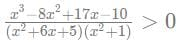Detailed Solution for Test: CAT Quantitative Aptitude- 3 - Question 13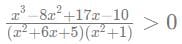Therefore, factorising the numerator, we get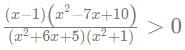Factorising the quadratic terms in the numerator and denominator, we get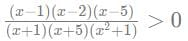In the denominator, x2 + 1 > 0, hence,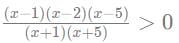The boundary points are x = -5, -1, 1, 2, 5
For x > 5, this inequality holds true.
Now, we know that if the powers of the terms are odd, the inequality alternates between them.
Hence, the inequality holds true for
x ∈ (−5, −1) U (1, 2) U (5, ∞)
Hence, integer values of variable x <= 10 satisfying the condition are -4, -3, -2, 6, 7, 8, 9, 10.
Hence, count = 8.

Test: CAT Quantitative Aptitude- 3 - Question 14

Cost of 1 pen, 3 pencils and 5 markers is Rs 100. Cost of 18 pencils, 2markers and 6 pens is Rs 320. What is the minimum possible cost of 1 marker, 1 pen and 1 pencils if it is given that the cost of each of the items is a natural number?

Detailed Solution for Test: CAT Quantitative Aptitude- 3 - Question 14

Let the cost of pen be xx, a pencil be y and a markers be z
x + 3y + 5z = 100.......(I)
6x + 18y + 2z = 320....(II)
6 × (I) − (II) gives us,
28z = 280 or z = 10
putting z = 10 in (I) we get x + 3y = 50
We have to get the minimum value of x + y + z. z = 10 thus we need to minimize x + y
when y = 16 then x = 2 and x + y + z = 2 + 16 + 10 = 28
When y = 15 then x = 5 and x + y + z = 5 + 15 + 10 = 30
We see that as we keep on decreasing y the sum will increase.
Minimum possible value = 28

*Answer can only contain numeric values
Test: CAT Quantitative Aptitude- 3 - Question 15

If t satisfies the following equation:
log3 ​78 + t − 6 = log3 ​(1 − 3t−5)
What is the value of |t|

Detailed Solution for Test: CAT Quantitative Aptitude- 3 - Question 15

Take t-5 = k
Equation modifies into
log3 78 + k − 1 = log3(1 − 3k)
Taking all the log terms to one side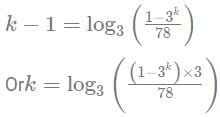Taking power 3 both sides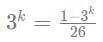Or 26 × 3k = 1 − 3k
27 × 3k =1
k = -3
Thus t - 5 = -3 or t = 2

*Answer can only contain numeric values
Test: CAT Quantitative Aptitude- 3 - Question 16

An entrepreneur borrows an amount of Rs 20 lakhs from a local bank for a duration of 3 years at a 20% interest rate, compounded annually. At the end of each of these 3 years, he returns a certain amount of money to the bank. The amount returned at the end of each of these 3 years is the same. At the end of 3 years, the bank additionally demanded a cheque of Rs. 1.8 lakhs to settle the loan. What was the amount he used to pay at the end of each year?

Detailed Solution for Test: CAT Quantitative Aptitude- 3 - Question 16

According to the question
2000000(1.2)3 = x(1.2)2 + x(1.2) + x + 180000
3456000 − 180000 = x(1.44 + 1.2 + 1)
3276000 = x(1.44 + 1.2 + 1)
3276000 = x × 3.6
3276000/​3.64 = x
x = 900000.

Test: CAT Quantitative Aptitude- 3 - Question 17

A milk merchant buys milk at Rs 50 per litre, but while selling, he marks up the price by 20% and also mixes water to the milk such that the milk-water mixture he sells has 80% milk and 20% water. Assuming that the water has zero cost price, what discount percentage should he give to the mixture such that by selling it, he earns a 10% profit?

Detailed Solution for Test: CAT Quantitative Aptitude- 3 - Question 17

CP of 1 litre = 50
MP of 1 litre = 1.2 x 50 = 60
Also, he mixes water to milk in the ratio of 1:4, so in 1 litre of milk, he mixes 250 mL of water.
Hence, the volume of liquid he is selling = 1.25
MP of 1.25 litres = 60 x 1.25 = 75 Rs.
So, to have a 10% profit, SP = Rs 50 x 1.1 = 55 Rs.
Hence, discount percent = 20/75 x 100 = 80/3%

*Answer can only contain numeric values
Test: CAT Quantitative Aptitude- 3 - Question 18

Ram was asked to calculate the sum of an arithmetic progression whose first term was 2 and common difference was 7. The total number of terms was 30. He wrote down all the 30 terms in ascending order and then started adding from smallest number. After calculating the sum of first x terms correctly, Ram started taking common difference as 14 instead of 7 and ended up with sum of 3210. How many factors does x − 4 has?

Detailed Solution for Test: CAT Quantitative Aptitude- 3 - Question 18

Let us calculate the actual sum which Ram would have got if he did not have had made the mistake.
We can use the formula to calculate the sum = 30/2 ​ (2(2) + (30 − 1)7)
Thus the actual sum = 15(4 + 29 × 7) = 3105
Excess sum calculated = 3210 - 3105 = 105
Let last n terms be the terms where the common difference was taken as 14 instead of 7
Thus 7 + 14 + ...n terms = 105
n/2(2(7) + (n − 1)7) = 105
n(n + 1)7 = 210
Or 2 + n − 30 = 0 or (n + 6)(n − 5) = 0.
Since n can not be negative, n = 5, Thus last 5 terms had incorrect common differece. x = 30 - n = 30 - 5 = 25
x − 4 = 21 = 3 × 7
Total 4 factors are there.

Test: CAT Quantitative Aptitude- 3 - Question 19

What is the number of common terms between the following series
A1: 4,10,16,22,28,..........,610
A2 ​: 2,9,16,23,............... 632

Detailed Solution for Test: CAT Quantitative Aptitude- 3 - Question 19

Both of the series are an Arithmetic Progression
Common difference of the Series are 6 and 7 respectively.
Upon looking, we can see that the first common term is 16.
The series which will have common terms will have first term as 16 and common difference = lcm(6, 7) = 42
Let an ​be the nth and final term of the series
an ​= 16 + (n − 1)42 ≤ 610
42(n − 1) = 594
n − 1 = 14.14 or n = 15.14. Thus their are total 15 number of common terms.

*Answer can only contain numeric values
Test: CAT Quantitative Aptitude- 3 - Question 20

X = Highest power of 144 in 25!
Y = Highest power of 96 in 50!
How many of the following sentences are correct about the value Y - X?
1. It is a perfect square
2. It is a perfect cube
3. It is prime
4. It is even

Detailed Solution for Test: CAT Quantitative Aptitude- 3 - Question 20

144 = 16 x 9 = 2432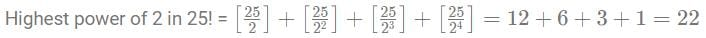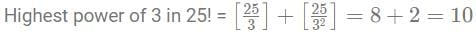Highest power of 16 = 5, highest power of 9 = 5.
Hence, highest power of 144 = 5.
96 = 32 x 3 = 253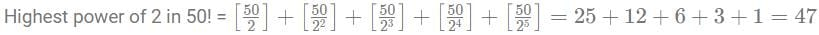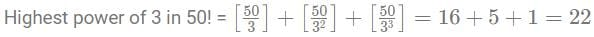Highest power of 32 = 9, highest power of 3 = 22.
Hence, the highest power of 96 = 9.
Y - X = 4. It is a perfect square and even.

Test: CAT Quantitative Aptitude- 3 - Question 21

A merchant purchases goods at the rate of 14 for Rs 105, marks them at the rate of 7 for Rs 140. If he offers 2 successive discounts on the marked price, d1% and d2%, such that d1 is one-fifth the markup percentage and d2 is half that of the value of d1, what is the profit/loss made by selling 90 goods?

Detailed Solution for Test: CAT Quantitative Aptitude- 3 - Question 21

CP of 1 good = 105/14 = Rs. 7.5
MP of 1 good = 140/7 = Rs. 20
Hence, markup % = 12.5/7.5 x 100 = 500/3 %
Hence, d1 = 100/3% = 1/3
d2 = 50/3% = 1/6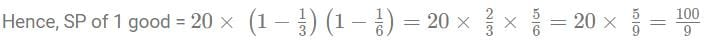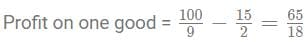Hence, profit on 90 goods = 65 x 5 = Rs 325.

*Answer can only contain numeric values
Test: CAT Quantitative Aptitude- 3 - Question 22

Amar drives his car twice the speed of an auto. One day, he drove the car for 10 min and the car broke mid-way. He left the car and took an auto to reach the office. It took him 30 min from there to reach the office. What would be the total time taken by car to reach his office?

Detailed Solution for Test: CAT Quantitative Aptitude- 3 - Question 22

Speed of the car is twice the speed of the auto. So, time taken by the car is half the time taken by the auto. Amar goes by car for 10 min and by auto for 30 min. Total time taken by him if he goes all the way be car = 10 + 1/2(30) = 10 + 15 = 25 min

## CAT Mock Test Series

2 videos|24 docs|89 tests
 Use Code STAYHOME200 and get INR 200 additional OFF Use Coupon Code
Information about Test: CAT Quantitative Aptitude- 3 Page
In this test you can find the Exam questions for Test: CAT Quantitative Aptitude- 3 solved & explained in the simplest way possible. Besides giving Questions and answers for Test: CAT Quantitative Aptitude- 3, EduRev gives you an ample number of Online tests for practice

## CAT Mock Test Series

2 videos|24 docs|89 tests

### How to Prepare for CAT

Read our guide to prepare for CAT which is created by Toppers & the best Teachers